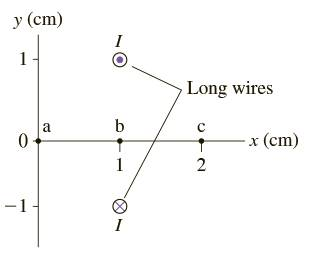Direction of Magnetic Fields

Homework StatementHow do I find the direction of the magnetic field at the points?

Homework Equations

Ampere's B = (UI) / (2*pi*r)[/B]

The Attempt at a Solution

I know how the Right hand rule, where your thumb is the direction of the current and your hand wraps the direction of the field, but I'm not sure how it plays in when both the current are opposite.

Try applying the right-hand rule to each of the currents separately and combining the results. Remember that fields add as vectors. and that the diagram is symmetric except for the directions of the currents.

Try applying the right-hand rule to each of the currents separately and combining the results. Remember that fields add as vectors. and that the diagram is symmetric except for the directions of the currents.
Yes , but aren't they equal and opposite? Wouldn't they just cancel each other?